TitleCollege Algebra
Answer/Discussion to Practice Problems
Tutorial 42: Exponential FunctionsAnswer/Discussion to 1a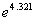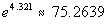Try this using your calculator and see if you get the answer that I got.  If you didn't, refer back to e key on calculator that I have above.Answer/Discussion to 1b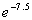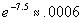Try this using your calculator and see if you get the answer that I got.  If you didn't, refer back to e key on calculator that I have above.Answer/Discussion to 2a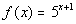Note that the base = 5 and the exponent is our variable x + 1.  There is one outside factor, we are adding 1 to our variable x in the exponent

I have found that the best way to do this is to do it the same each time.  In other words, put in the same values for x each time and then find it's corresponding y value for the given function.

 x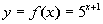(x, y) -2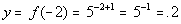(-2, .2) -1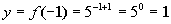(-1, 1) 0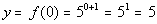(0, 5) 1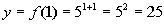(1, 25) 2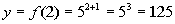(2, 125)

 Step 2: Plot points. AND Step 3: Draw curve.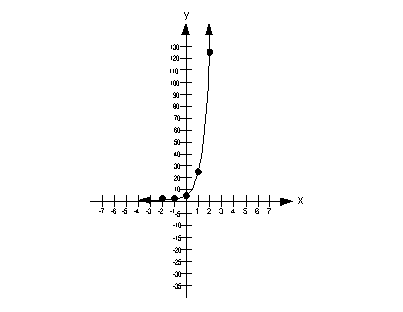Answer/Discussion to 2bNote that the base = 1/5 and the exponent is our variable x.  There are 2 outside factors, we are multiplying it by -5  AND we are adding 2 to our base after we raise it to the exponent x and multiply that by -5.

I have found that the best way to do this is to do it the same each time.  In other words, put in the same values for x each time and then find it's corresponding y value for the given function.

 x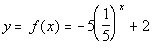(x, y) -2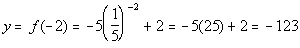(-2, -123) -1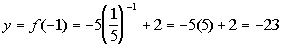(-1, -23) 0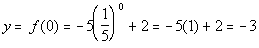(0, -3) 1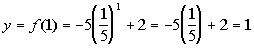(1, 1) 2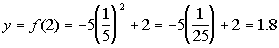(2, 1.8)

 Step 2: Plot points. AND Step 3: Draw curve.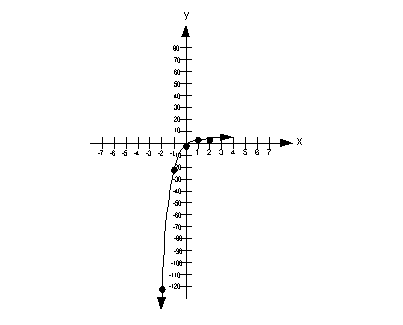Answer/Discussion to 3a \$7500 for 25 years at an annual rate of 4.25% compounded monthly.

 P = 7500 r = 4.25% = .0425 t = 25 n = monthly = 12 times a year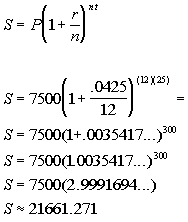*Plug in values shown above into compound form. *Find number inside the (  ) first *Raise the (  )  to the 300th power *Multiply

 So the compound AMOUNT would be \$21661.27 The compound amount is the total amount that is in the account. How do you think we are going to get the interest?? Well we have the principle which is the beginning amount and we have the compound amount which is the end result. Looks like, if we take the difference of the two, that will give us how much interest was earned from beginning to end. What do you think?  Compound amount - principle: 21661.27 - 7500 = 14161.27 So our compound interest is \$14161.27Answer/Discussion to 3b \$3000 for 20 years at an annual rate of 6% compounded quarterly.
 P = 3000 r = 6% = .06 t = 20 n = quarterly = 4 times a year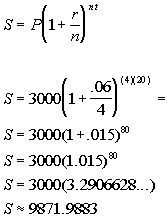*Plug in values shown above into compound form. *Find number inside the (  ) first *Raise the (  )  to the 300th power *Multiply

 So the compound AMOUNT would be \$9871.99 The compound amount is the total amount that is in the account. How do you think we are going to get the interest?? Well we have the principle which is the beginning amount and we have the compound amount which is the end result. Looks like, if we take the difference of the two, that will give us how much interest was earned from beginning to end. What do you think?  Compound amount - principle: 9871.99 - 3000 = 6871.99  So our compound interest is \$6871.99Answer/Discussion to 4a \$8000 that is compounded continuously for 10 years at an interest rate of 5.5%.

 P =  8000 r = 5.5% = .055 t = 10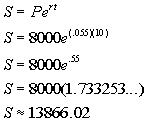*Plug in values shown above into compound form. *Raise e to the .55th power *Multiply

 So the accumulated or compound AMOUNT would be \$13866.02.

Last revised on March 17, 2011 by Kim Seward.
All contents copyright (C) 2002 - 2011, WTAMU and Kim Seward. All rights reserved.